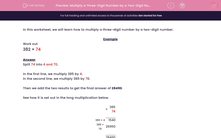# Multiply a Three-Digit Number by a Two-Digit Number

In this worksheet, students use long multiplication to find the product of a three-digit number and a two-digit number.Key stage:  KS 2

Curriculum topic:   Maths and Numerical Reasoning

Curriculum subtopic:   Mixed Problems

Difficulty level:#### Worksheet Overview

In this worksheet, we will learn how to multiply a three-digit number by a two-digit number.

Example

Work out

382 × 74

Split 74 into 4 and 70.

In the first line, we multiply 385 by 4.

In the second line, we multiply 385 by 70.

Then we add the two results to get the final answer of 28490.

See how it is set out in the long multiplication below.

 × 385 74 385 × 4 1540 385 × 70 26950 28490

### What is EdPlace?

We're your National Curriculum aligned online education content provider helping each child succeed in English, maths and science from year 1 to GCSE. With an EdPlace account you’ll be able to track and measure progress, helping each child achieve their best. We build confidence and attainment by personalising each child’s learning at a level that suits them.

Get started